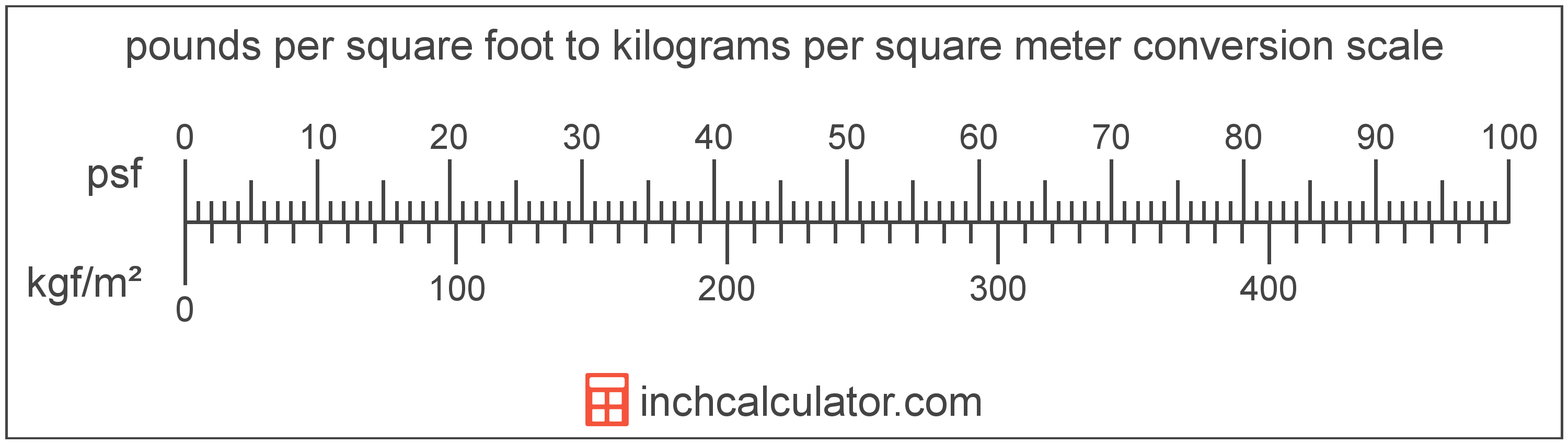# Pounds per Square Foot to Kilograms per Square Meter Converter

Enter the pressure in pounds per square foot below to get the value converted to kilograms per square meter.

Results in Kilograms per Square Meter:1 psf = 4.882422 kgf/m²

Do you want to convert kilograms per square meter to pounds per square foot?

## How to Convert Pounds per Square Foot to Kilograms per Square Meter

To convert a measurement in pounds per square foot to a measurement in kilograms per square meter, multiply the pressure by the following conversion ratio: 4.882422 kilograms per square meter/pound per square foot.

Since one pound per square foot is equal to 4.882422 kilograms per square meter, you can use this simple formula to convert:

kilograms per square meter = pounds per square foot × 4.882422

The pressure in kilograms per square meter is equal to the pressure in pounds per square foot multiplied by 4.882422.

For example, here's how to convert 5 pounds per square foot to kilograms per square meter using the formula above.
kilograms per square meter = (5 psf × 4.882422) = 24.412112 kgf/m²Pounds per square foot and kilograms per square meter are both units used to measure pressure. Keep reading to learn more about each unit of measure.

## What Are Pounds per Square Foot?

One pound per square foot is the pressure of equal to one pound-force per square foot.

The pound per square foot is a US customary and imperial unit of pressure. Pounds per square foot can be abbreviated as psf; for example, 1 pound per square foot can be written as 1 psf.

PSF can be expressed using the formula:
1 psf = 1 lbf / ft2

Pressure in pounds per square foot are equal to the pound-force divided by the area in square feet.

## What Are Kilograms per Square Meter?

One kilogram per square meter is the pressure of equal to one kilogram-force per square meter.

The kilogram per square meter is a non-SI metric unit for pressure. A kilogram per square meter is sometimes also referred to as a kilogram per square metre or kilogram-force per meter square. Kilograms per square meter can be abbreviated as kgf/m²; for example, 1 kilogram per square meter can be written as 1 kgf/m².

In the expressions of units, the slash, or solidus (/), is used to express a change in one or more units relative to a change in one or more other units. For example, kgf/m² is expressing a change in weight relative to a change in area.

The unit is deprecated and not permitted for use with SI units.

Kilograms per square meter can be expressed using the formula:
1 kgf/m2 = 1 kgf / m2

Pressure in kilograms per square meter are equal to the kilogram-force divided by the area in square meters.

## Pound per Square Foot to Kilogram per Square Meter Conversion Table

Table showing various pound per square foot measurements converted to kilograms per square meter.
Pounds Per Square Foot Kilograms Per Square Meter
1 psf 4.8824 kgf/m²
2 psf 9.7648 kgf/m²
3 psf 14.65 kgf/m²
4 psf 19.53 kgf/m²
5 psf 24.41 kgf/m²
6 psf 29.29 kgf/m²
7 psf 34.18 kgf/m²
8 psf 39.06 kgf/m²
9 psf 43.94 kgf/m²
10 psf 48.82 kgf/m²
11 psf 53.71 kgf/m²
12 psf 58.59 kgf/m²
13 psf 63.47 kgf/m²
14 psf 68.35 kgf/m²
15 psf 73.24 kgf/m²
16 psf 78.12 kgf/m²
17 psf 83 kgf/m²
18 psf 87.88 kgf/m²
19 psf 92.77 kgf/m²
20 psf 97.65 kgf/m²
21 psf 102.53 kgf/m²
22 psf 107.41 kgf/m²
23 psf 112.3 kgf/m²
24 psf 117.18 kgf/m²
25 psf 122.06 kgf/m²
26 psf 126.94 kgf/m²
27 psf 131.83 kgf/m²
28 psf 136.71 kgf/m²
29 psf 141.59 kgf/m²
30 psf 146.47 kgf/m²
31 psf 151.36 kgf/m²
32 psf 156.24 kgf/m²
33 psf 161.12 kgf/m²
34 psf 166 kgf/m²
35 psf 170.88 kgf/m²
36 psf 175.77 kgf/m²
37 psf 180.65 kgf/m²
38 psf 185.53 kgf/m²
39 psf 190.41 kgf/m²
40 psf 195.3 kgf/m²

## References

1. National Institute of Standards and Technology, NIST Guide to the SI, Chapter 6: Rules and Style Conventions for Printing and Using Units, https://www.nist.gov/pml/special-publication-811/nist-guide-si-chapter-6-rules-and-style-conventions-printing-and-using